Sklansky 1982

In 1982 Sklansky presented a revised version  of his original algorithm.

The new algorithm consisted of two parts. Part 1 was inteded to take a simple polygon and return a maximal polygon (monotonic in both the horizontal and vertical directions, see figure below). Part 2 was Sklansky's original algorithm, which was guarranteed to work on the output of part 1, since maximal polygons are weakly externally visible, and it is proven that Sklansky's original algorithm works for such polygons .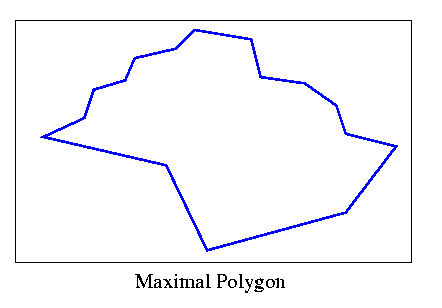Sklansky's idea was good. He presented a relatively simple procedure which would ensure that his previous elegant algorithm would work. It could even be argued that the entire process would be "simpler" than that of McCallum and Avis (their algorithm used two stacks, while this one used two stages, so it's a subjective call). Unfortunately, Sklansky's revised algorithm didn't escape the fate of the original. It was proven wrong by Toussaint just a few months later .

I provide the main idea of part 1 below. Anyone who is interested in examining the original source should be warned that on page 81 the third line from the top should read "yi+2-yi+1<=0, retain Vi+2 in P" instead of "yi+2-yi<0, retain Vi+1 in P", as pointed out by Toussaint.

The first thing that Sklansky does is find the extreme vertices in the horizontal and vertical directions. Then he separates the plane as shown below: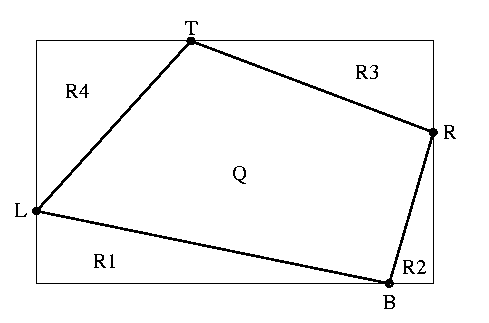T, B, L, R refer to the top, bottom, left and right extreme vertices. All points of the simple polygon are within the rectangle. The idea is to create four monotone chains within the regions Ri.

Sklansky demonstrates how this is to be done for R1. Suppose that two vertices I, I+1 have been accepted onto the monotone chain beginning at L. Separate the plane into three regions as shown below.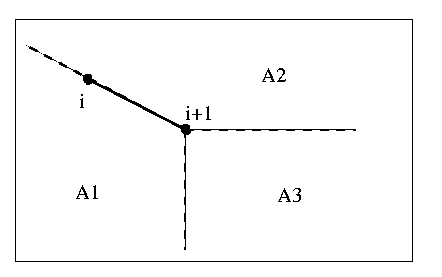The next vertex, I+2 is handled as follows:

1)    If I+2 is is not in R1, discard I+2.

2)    Else if I+2 is in A3, retain I+2 and re-iterate.

3)    Else if (I+2 is in A2) OR (I+2 is in A1 AND I+2 is above I+1 AND a vertex was discarded due to line 3 on the immediately preceeding iteration), discard I+2.

4)    Else if I+2 is in A1, discard I+1.

Finally, if line 3 is carried out, I+2 takes the role of I+1 in the next iteration, and if line 4 is carried out, vertex (I) takes the role of I+1.

It seems a little strange to me that even though vertices may be discarded, they are used for further calculations. This may violate the original assumption that vertices I, I+1 have been accepted.

It is quite easy to create a chain which will not become monotone when this algorithm is applied. In the figure below, the algorithm will advance and retain all vertices until vertex V becomes I+2 and is deleted by line 3. Then V will take on the role of I+1, and all the vertices further on are in region A3. Therefore, the only vertex removed is V, and the resulting chain is not monotone in the vertical direction.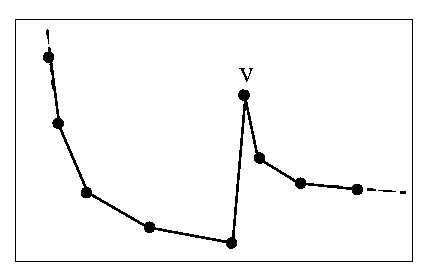Toussaint pointed out a couple of counterexamples to the algorithm which are shown below. Note that all points are within region R1.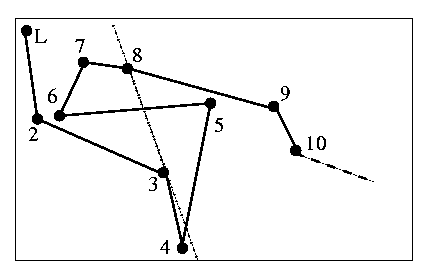For the above chain, Sklansky's algorithm yields the chain L,2,3,4,8,9,10...B, which is non-simple.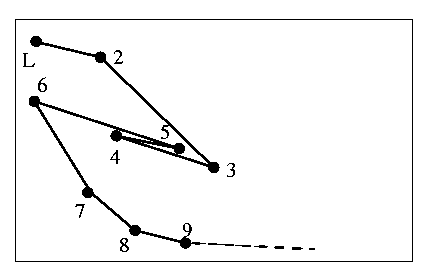In this case, the algorithm yields the chain L,2,7,8,9...B. Vertex 6 which is on the convex hull, has been discarded.

Toussaint also states that it is possible to create situations where regions A1,A2,A3 are undefined.

previous : Kim 1980.          next : Lee 1983.